##1. Parallel trilinear polars

 Every conic passing through the vertices of the triangle of reference ABC and the centroid G of this triangle is a hyperbola.
 All trilinear polars tr(E) of points E lying on such a conic are parallel to each other and define a point at infinity P0.
 P0 is the perspector of the hyperbola with respect to the triangle ABC.
 The trilinear polar L=tr(P0) of P0 (the perspectrix of the hyperbola) is the diameter of the hyperbola which is conjugate to the common direction of all tr(E). L passes through the center of the hyperbola and is tangent to the inner Steiner ellipse of the triangle the tangency point coinciding with the center of the hyperbola.
 Line L is also the isotomic dual of the tangent tG of the hyperbola at the centroid G.
 The isotomic t(P0) of P0 is on the outer Steiner ellipse and the centroid G is on the conjugate diameter of tG.
 The line through {G,t(P0)} intersects the hyperbola at a second point G' which is symmetric to t(P0) with respect to G. G' coincides with the tripole tr(GP0) of line GP0.
 The parallelogram with vertices {t(P0),S1,G',S2}, where {S1,S2} are the intersections of ~P0 with the outer Steiner ellipse, has its sides parallel to the asymptotes of the hyperbola. Hence these are parallel to conjugate diameters of the Steiner ellipse.
 Inversely every conic having its perspector P0 at infinity has all the above properties.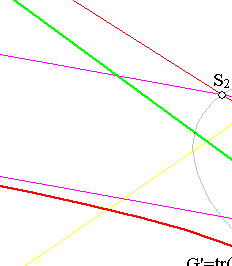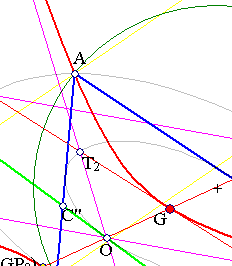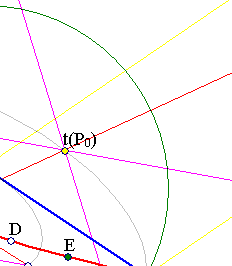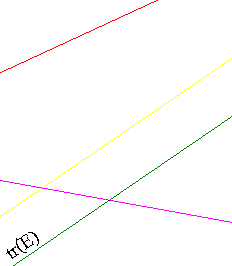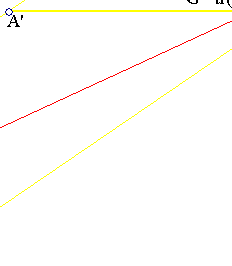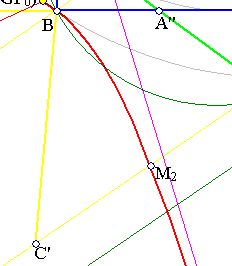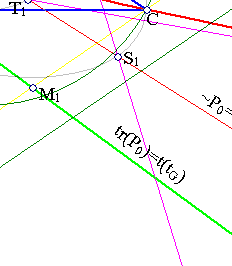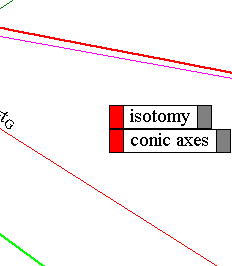The proof is trivial in barycentric coordinates. In fact, the conic through {A,B,C} has an equation of the form
a/x + b/y + c/z = 0.
If G(1,1,1) is on the conic then a+b+c=0, which means that P0(a,b,c) is a point at infinity (see Trilinears.html ) and by its definition it is the perspector of the conic.
On the other side the trilinear polar of a point X(u,v,w) on the conic is
x/u + y/v + z/w = 0.
But a/u + b/v + c/w = 0 implies that this line passes through P0. This proves  and .
That this conic is actually a hyperbola follows fromt the fact that it is the isotomic image of line ax+by+cz=0 which passes through G. Thus it intersects the outer Steiner ellipse at two distinct points, which by isotomy map to points at infinity. This shows .
 Is a consequence of the discussion in HyperbolaPropertyParallels.html where the construction of the trilinear polar of a point at infinity is carried out. To find the trilinear polar L=tr(P0) where P0 is the point at infinity represented by the common direction of all tr(E) the following constructions are used:
(i) Draw parallels to tr(E) from the vertices of ABC.
(ii) Find the intersections {A',B',C'} of these parallels with the opposite sides of ABC.
(iii) Take the harmonic conjugates {A'',B'',C''} of the former points with respect to {(B,C),(C,A),(A,B)} respectively.
Line L=tr(P0) coincides with the line containing the points {A'',B'',C''}.
Since L is the polar of the point at infinity P0 the lines through that point intersect the conic in segments having their middles on L (in the figure the middle of CM2 is M1). This proves .
 follows from a trivial calculation showing that line ax+by+cz=0, containing G(1,1,1), is tangent at to the conic at G. Since this line maps through isotomy to the conic it is the dual of the perspectrix tr(P0) (see IsotomicConicOfLine.html ).
To show property  use the previously proved tG = ~P0 = tr(t(P0)). Hence tr(tG) = tr(tr(t(P0))) = t(P0). This has coordinates (barycentric) (1/a,1/b,1/c) hence the line through G and t(P0) has the form: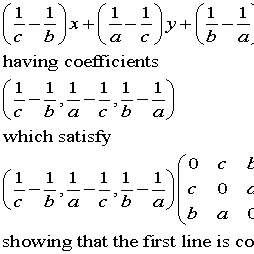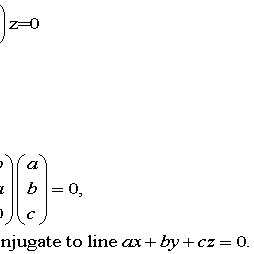The matrix is the one expressing the conic as a quadratic form. The claim in  follows from this matrix identity.
To prove  it suffices to express G'=t(P0)+sG, then calculate s by applying the equation of the conic to G':
q(G',G')=0, q(X,Y) denoting the quadratic form expressing the conic through the matrix considered above.
Replacing the equation one calculates easily that s = (a2+b2+c2)/(a3+b3+c3).
Express now G in terms of G' and t(P0): G = (1/t)(G'-t(P0). To show the claim it suffices to show that the harmonic conjugate of G with respect to {G',t(P0)}, which is (2t(P0)+sG) is a point at infinity (i.e. satisfies x+y+z=0).
A short calculation reduces this to showing that a3+b3+c3=3abc. But this is satisfied by every tripple for which a+b+c=0.
To show  let {S1,S2} be the intersection points of line ~P0 with the outer Steiner ellipse. Since the outer Steiner ellipse is the isotomic image of the line at infinity these points have isotomic conjugates {t(S1),t(S2)} the intersection points of the hyperbola with the line at infinity.
Hence lines S1t(S1) and S2t(S2) are parallel to the asymptotes of the hyperbola.
These lines pass also through t(P0).
To show this consider the barycentric coordinates of S1, (x,y,z) say. Since this is a point of ~P0 it satisfies
ax+by+cz=0. (1)
Since it is also on the outer Steiner ellipse it satisfies (1/x) + (1/y) + (1/z) = 0. (2)
In addition we have a+b+c = 0. (3)
The collinearity stated for line S1t(S1) is equivalent with the vanishing of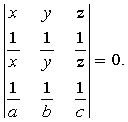Solving (1) and (3) with respect to (a,b,c) we find:
(a,b,c) = ( y-z , z-x , x-y ). (4)
Introducing this into the above determinant and expanding it we get the equivalent equation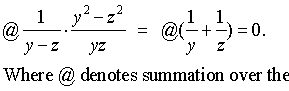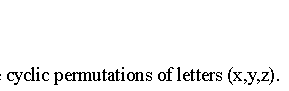Last equation is a consequence of (2). This proves the claim for line S1t(S1) and analogously goes the proof for the other line.
To show  consider the tripols of lines passing through P0. Among these lines is the line at infinity having for tripole the centroid. Besides all these tripols are points of the conic (see IsotomicConicOfLine.html ). This implies that  is valid, from which all other properties follow.

##2. Remarks

 In HyperbolaPropertyParallels.html was shown that CM1 is (1/3) of CC'. Hence (in view of  above) M1, M2 divide CC' in three equal parts. This implies an easy construction of a fifth point (M2) of the hyperbola once the direction of tr(E) is given. Analogous additional points of the hyperbola exist on lines AA' and BB'.
 The figure shows the two Steiner ellipses. Points t(P0) being isotomic to points at infinity are on the outer Steiner ellipse. On the other side G' being symmetric to t(P0) with respect to G it is also on the outer Steiner ellipse.
 Once the direction of tr(E) is known the center of the conic is easily constructible trhough the intersection of lines Gt(P0) and tr(P0). Since G, G' are symmetric with respect to O later is on the inner Steiner ellipse hence it is the contact point of the line tr(P0) with that conic.
 Consider the intersection points {T1,T2} of the asymptotes with ~P0. Triangles OT1T2 and t(P0)S2S1 are anti-homothetic with respect to G, hence {T1,T2} are also on the inner Steiner ellipse.
 By the remark 2.6 in IsotomicConicOfLine.html the trilinear polars of the points at infinity of the hyperbola will be tangent to the inner Steiner ellipse and so that the chord of contacts is the polar of P0 which is ~P0. Thus these lines are the tangents to the inner Steiner ellipse at points {T1,T2}.
 The most prominent member of this family of conics (passing through G) is the Kiepert hyperbola passing also through the orthocenter. Its tangent at G contains the symmedian point K of the triangle. Passing through the orthocenter implies it is a rectangular hyperbola, hence, in view of , its asymptotes are parallel to the axes of the Steiner ellipse.

##3. Generalizing

The subject studied here is essentially the investigation of the geometric properties of all conics passing through four particular points {A,B,C,G}, which form a triangle together with its centroid. One could fix the three first points, select another fourth point S instead of G and study the geometric properties of the resulting family of conics passing through the four points {A,B,C,S}. This is done in ConicsThroughFourGeometric.html .

ConicsThroughFourGeometric.html
Desargues.html
Harmonic.html
Harmonic_Bundle.html
HyperbolaPropertyParallels.html
IsotomicConicOfLine.html
LineInTrilinears.html
TriangleConics.html
Trilinears.html
TrilinearPolar.html
Tripole.html